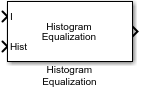# Histogram Equalization

Enhance contrast of images using histogram equalization

•Libraries:
Computer Vision Toolbox / Analysis & Enhancement

## Description

The Histogram Equalization block enhances the contrast of images. The block transforms the intensity values in an image so that the histogram of the output image approximately matches a specified histogram. By default, the block transforms the input image so that the histogram of the output image is nearly flat.

## Ports

### Input

expand all

Input image, specified as a matrix of intensity values.

Data Types: `single` | `double` | `int8` | `int16` | `int32` | `uint8` | `uint16` | `uint32` | `fixed point`

Target histogram for the output image, specified as a vector of integers. Each element of the vector represents the desired count value for the corresponding bin. You must normalize the input vector such that the sum of all the bin counts equals the number of pixels in the input image.

When the data type of the input image is `single` or `double`, the Hist port input must be the same data type.

#### Dependencies

To enable this port, set these block parameters:

• Target histogram: `User-defined`

• Histogram source: ```Input port```

Data Types: `single` | `double` | `int8` | `int16` | `int32` | `uint8` | `uint16` | `uint32`

### Output

expand all

Output image, returned as a matrix of intensity values. The data type of the output image is the same as the data type of the input image.

Data Types: `single` | `double` | `int8` | `int16` | `int32` | `uint8` | `uint16` | `uint32` | `fixed point`

## Parameters

expand all

Specify the desired histogram type.

• `Uniform` — The block transforms the input image so that the histogram of the output image is approximately flat.

• `User-defined` — The block transforms the input image so that the histogram of the output image approximately matches the specified histogram.

Specify the number of bins for the target histogram. The block spaces the bins equally.

#### Dependencies

To enable this parameter, set the Target histogram parameter to `Uniform`.

Specify the source of the target histogram as either ```Specify via dialog``` or `Input port`.

• `Specify via dialog` — Use the Histogram parameter to specify a vector of integer values representing the desired counts of the equally spaced bins of the target histogram.

• `Input port` — Use the Hist input port to specify the target histogram.

#### Dependencies

To enable this parameter, set the Target histogram parameter to `User-defined`.

Note

Normalize the input vector, specified using the selected Histogram source, such that the sum of all the bin counts equals the number of pixels in the input image. The block does not display an error if you do not normalize the vector.

Specify the count for each bin of the target histogram. The sum of the bin counts must equal the number of pixels in the input image.

#### Dependencies

To enable this parameter, set these block parameters:

• Target histogram: `User-defined`

• Histogram source: ```Specify via dialog```

## Block Characteristics

 Data Types `double` | `fixed point` | `integer` | `single` Multidimensional Signals `no` Variable-Size Signals `no`

 Gonzalez, Rafael C., and Richard E. Woods. Digital Image Processing: International Edition. 3rd ed. Upper Saddle River, NJ: Pearson, 2008.